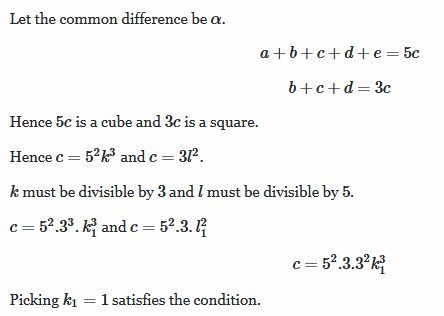# Let a,b,c,d and e be in the A.P such that a+b+c+d+e is the cube of an integer and b+c+d is square of an integer. Then the least possible value of c is

Hi,
If m,n,o are in AP then m+o = 2n
so sum of m,n,o = m+n+o = 2n+n = 3n
so basically 3 times the middle number
same concept will be used for 4 AP numbers or 5 AP numbers ,etcSo number c= 675

• -1
What are you looking for?